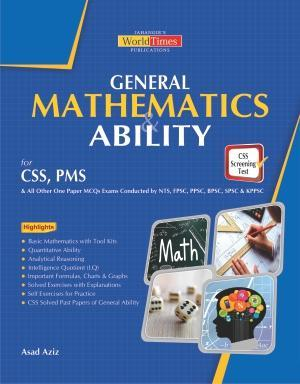Friday , January 27 2023
Home / Archive / Types of Sets By Sir Asad Aziz

# Types of Sets By Sir Asad Aziz

1. ____, universal, proper, singleton, and power sets are a few of the many types of sets.
(a) Finite             (b) Infinite
(c) Subset           (d) All of the above

2. Whenever n is a non-negative integer, a set is said to be finite if it contains only _____ distinct elements.
(a) n                (b) n+1
(c) n-1              (d) n^2

3. An ____ set is a finite set.
(a) Innumerable              (b) Enumerable
(c) Both (a) and (b)          (d) None of these

4. A/an ____ set is one that has no end.
(a) Non-finite                 (b) Finite
(c) Infinite                     (d) None of these

5. The ____ is n.
(a) Cardinality of sets      (b) Cardinal number of sets

(c) Both (a) and (b)       (d) None of these

6. ______ is another name for a countably infinite set.
(a) Enumerable             (b) Denumerable
(c) Non-numerable       (d) Innumerable

7. In mathematics, countable sets are defined as ______.
(a) Finite                        (b) Denumerable
(c) Both (a) and (b)     (d) None of these

8. A non-negative even integer set is ______.
(a) Countable Infinite       (b) Uncountable Finite
(c) Countable Finite       (d) Uncountable Infinite

9. Infinite sets that can’t be counted are called _____.
(a) Uncountable infinite sets   (b) Non-denumerable sets
(c) Uncountable sets                (d) All of the above

10. If every element of A is also an element of B, then ______.
(a) A is called Superset of B      (b) B is called Superset of A
(c) A and B are Superset           (d) B is called Subset of A

11. A is called a subset of B, if ______ element of A is also an element of B.
(a) Few             (b) None
(c) Little            (d) Every

12. If every element of A is also an element of B, it is denoted by:
(a) A ⊆ B         (b) A U B
(c) A – B            (d) A + B

13. Which of the following is/are a property (properties) of a subset?
(a) Sets are subsets of one another.
(b) Every set contains a Null Set, i.e.∅.
(c) The subset of A will be C if it is a subset of B and B is a subset of C.
(d) All of the above14. Which of the following is a property of a subset?
(a) If there are n elements in a finite set, there are 2^n subsets
(b) If there are n elements in a finite set, there are n^2 subsets
(c) If there are n elements in an infinite set, there are 2^n subsets
(d) If there are n elements in an infinite set, there are n^2 subsets

15. If A⊂B and B⊂ C ⟹ ____
(a) C ⊂ A           (b) A ⊂ C
(c) A U C           (d) A + C

16. Whenever A is a subset of B, and A ≠ B, then A is called a ___ subset of B.
(a) Proper       (b) Improper
(c) Finite           (d) Infinite

17. B ____ of A if A is a proper subset of B.
(a) Is not a subset         (b) Is a subset
(c) Is not a superset        (d) None of these

18. Every set has a null ____ as a proper subset.
(a) =           (b) ∅
(c) *            (d) U

19. Sets are ____ subsets of themselves.
(a) Proper       (b) Improper
(c) Finite         (b) Infinite

20. ____ sets, do not contain any elements.
(a) Null             (b) Void
(c) Empty         (d) All of the above

## The Looming Debt Default

Listen to this article The Looming Debt Default Emerging economies are facing a high risk …